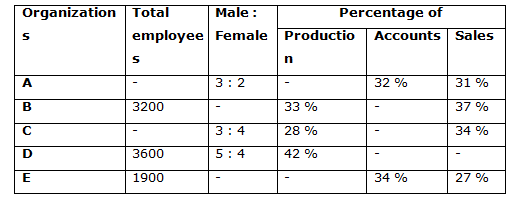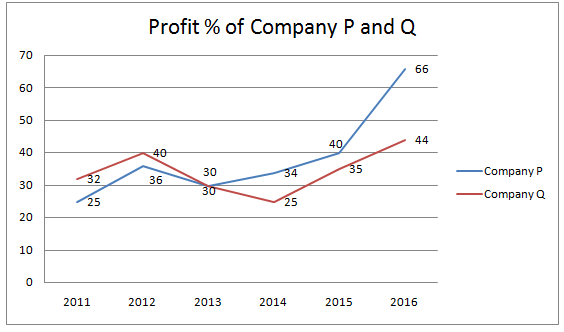# Quantitative Aptitude Questions (Data Interpretation) for NIACL Assistant Mains 2018 Day-188

Dear Readers, IBPS NIACL is conducting Online Examination for the recruitment of Assistant. To enrich your preparation here we have providing new series of Data Interpretation – Quantitative Aptitude Questions. Candidates those who are appearing in NIACL Assistant Mains Exams can practice these Quantitative Aptitude average questions daily and make your preparation effective.

[WpProQuiz 3548]

Click “Start Quiz” to attend these Questions and view Solutions

Directions (1 – 5): Study the following information carefully and answer the given questions:

The following table shows the total number of employees in 5 different organizations and the ratio of male and female among them and the percentage distribution of total employees in production, Accounts and sales department.1) Find the average number of employees in all the given organizations together, if the total number of males in organization A is 1500 and the total employees in production department in organization C is 784?

a) 2600

b) 2750

c) 2800

d) 2850

e) None of these

2) Total number of employees in production department in organization A and E together is approximately what percentage of total number of employees in Accounts department in organization B and D together, if percentage distribution of sales department in organization D is 32 % and the total number of employees in sales department in organization A is 775?

a) 88 %

b) 75 %

c) 100 %

d) 65 %

e) 120 %

3) Find the ratio between the total number of male employees in organization C to that of total number of female employees in organization D, if the total number of female employees in organization C is 1600?

a) 5 : 6

b) 1 : 2

c) 7 : 9

d) 3 : 4

e) None of these

4) Find the difference between the total number of employees in Accounts and sales department together in organization B to that of D?

a) 78

b) 56

c) 62

d) 90

e) None of these

5) Total employees in organization A and E together is approximately what percentage more/less than the total employees in organization B and D together, if the total number of female employees in organization A is 1000?

a) 35 % less

b) 25 % more

c) 25 % less

d) 35 % more

e) 15 % more

Directions (6 – 10): Study the following information carefully and answer the given questions:

The following line graph shows the percentage of profit earned by two different companies during the year 2011 to 2017.6) If the incomes of company P and Q are same in the year 2012, then find the ratio between the expenditures of company P to that of Q?

a) 15 : 13

b) 21 : 17

c) 35 : 34

d) 42 : 37

e) None of these

7) If the income of company P in 2011 and the expenditure of company Q in 2014 is same, which is equal to 65 lakhs, then find the difference between the expenditure of company P in 2011 to that of the income of company Q in 2014?

a) 29.25 lakhs

b) 31.75 lakhs

c) 33 lakhs

d) 27.5 lakhs

e) None of these

8) Find the ratio between the profit % of company P to that of Q in all the given years?

a) 152 : 137

b) 314 : 301

c) 78 : 55

d) 231 : 206

e) None of these

9) If the income of company Q in 2014 and the expenditure of company P in 2012 is 72 lakhs and 56 lakhs respectively, then find the sum of the expenditure of company Q in 2014 and the income of company P in 2012?

a) 152 lakhs

b) 76 lakhs

c) 145.25 lakhs

d) 138.34 lakhs

e) None of these

10) The expenditure of company P in 2015 is approximately what percentage of the income of company P in same year?

a) 52 %

b) 64 %

c) 71 %

d) 83 %

e) 45 %

Direction (1-5) :

The total number of males in organization A = 1500

The total employees in organization A = (1500/3)*5 = 2500

The total employees in production department in organization C = 784

(28/100)*total employees in organization C = 784

The total employees in organization C = 784*(100/28) = 2800

The average number of employees in all the given organizations together

= > [2500 + 3200 + 2800 + 3600 + 1900]/5

= > 14000/5 = 2800

The percentage distribution of sales department in organization D = 32 %

Total employees in Accounts department in organization D

= > 3600*(26/100) = 936

The total number of employees in sales department in organization A = 775

= > (31/100)*total employees in organization A = 775

= > Total employees in organization A = 775*(100/31) = 2500

Total number of employees in production department in organization A and E together

= > 2500*(37/100) + 1900*(39/100)

= > 925 + 741 = 1666

Total number of employees in Accounts department in organization B and D together

= > 3200*(30/100) + 936

= > 960 + 936 = 1896

Required % = (1666/1896)*100 = 87.8 % = 88 %

The total number of female employees in organization C = 1600

The total number of male employees in organization C

= > (1600/4)*3 = 1200

The total number of female employees in organization D

= > 3600*(4/9) = 1600

Required ratio = 1200 : 1600 = 3 : 4

The total number of employees in Accounts and sales department together in organization B

= > 3200*(67/100) = 2144

The total number of employees in Accounts and sales department together in organization D

= > 3600*(58/100) = 2088

Required difference = 2144 – 2088 = 56

The total number of female employees in organization A = 1000

= > (2/5)*total employees in organization A = 1000

= > Total employees in organization A = 1000*(5/2) = 2500

The total employees in organization A and E together

= > 2500 + 1900 = 4400

The total employees in organization B and D together

= > 3200 + 3600 = 6800

Required % = [(6800 – 4400)/6800]*100 = 35 % less

Direction (6-10) :

Let the incomes of company P and Q in the year 2012 be x,

The expenditures of company P = x*(100/136)

The expenditures of company Q = x*(100/140)

Required ratio = [x*(100/136)] : [x*(100/140)] = 35 : 34

The income of company P in 2011 = 65 lakhs

The expenditure of company P in 2011 = 65*(100/125) = 52 lakhs

The expenditure of company Q in 2014 = 65 lakhs

The income of company Q in 2014 = 65*(125/100) = 81.25 lakhs

Required difference = 81.25 – 52 = 29.25 lakhs

The profit % of company P in all the given years

= > 25 + 36 + 30 + 34 + 40 + 66 = 231

The profit % of company Q in all the given years

= > 32 + 40 + 30 + 25 + 35 + 44 = 206

Required ratio = 231 : 206

The income of company Q in 2014 = 72 lakhs

The expenditure of company Q in 2014 = 72*(100/125) = 57.6 lakhs

The expenditure of company P in 2012 = 56 lakhs

The income of company P in 2012 = 56*(136/100) = 76.16 lakhs

Required sum = 57.6 + 76.16 = 133.76 lakhs

Let the expenditure of company P in 2015 be x,

The income of company P in 2015 = x*(140/100) = 7x/5

Required % = [x/(7x/5)]*100 = 71.4 % = 71 %

Daily Practice Test Schedule | Good Luck

 Topic Daily Publishing Time Daily News Papers & Editorials 8.00 AM Current Affairs Quiz 9.00 AM Quantitative Aptitude “20-20” 11.00 AM Vocabulary (Based on The Hindu) 12.00 PM General Awareness “20-20” 1.00 PM English Language “20-20” 2.00 PM Reasoning Puzzles & Seating 4.00 PM Daily Current Affairs Updates 5.00 PM Data Interpretation / Application Sums (Topic Wise) 6.00 PM Reasoning Ability “20-20” 7.00 PM English Language (New Pattern Questions) 8.00 PM